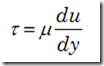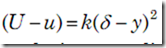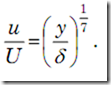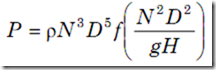### Anna University - MECHANICS OF FLUIDS (MOF)–April / May 2010 Question Paper

B.E./B.Tech. DEGREE EXAMINATION, APRIL/MAY 2010

Third Semester

Civil Engineering

CE2202 — MECHANICS OF FLUIDS (Regulation 2008)

Time: Three hours

Maximum: 100 Marks

PART A — (10 * 2 = 20 Marks)

1. Define the term continuum.

2. What is the difference between an ideal and a real fluid?

3. Distinguish between path lines, stream lines and streak lines.

4. To what type of flow is the concept of velocity potential and stream function applicable?

5. What are the assumptions made in the derivation of Euler's equation?

6. Sketch the velocity and shear stress distribution for laminar flow of an incompressible fluid through a circular pipe.

7. Give four examples in every day life where formation of boundary layer is important.

8. What are the characteristics of laminar flow?

9. What are the applications of model testing?

10. Enumerate the applications of dimensional homogeneity.

PART B — (5 * 16 = 80 Marks)

11. (a) (i) An open reservoir contains a liquid having density of 1.23 g/cc. At a certain point the gauge pressure is 0.31 atmosphere. At what height above the given point is the liquid level? (8)

(ii) Define Viscosity. Explain the effect of temperature and pressure on viscosity of liquids and gases. (8)

Or

(b) (i) Explain the characteristics of non- Newtonian fluids in detail. (8) (ii) The velocity distribution for flow over a plate is given by u = 2 y – y2 where u is the velocity in m/s at a distance y meters above the plate. Determine the velocity gradient and shear stress at the boundary and 0.15 m from it. (8)

12. (a) Derive an expression for the depth of centre of pressure from free surface of liquid of an inclined plane surface submerged in the liquid. (16)

Or

(b) (i) Derive the differential equation of continuity. (8)

(ii) In a two dimensional incompressible flow, the fluid velocity

components are given by

u = x - 4 y and

v = - y - 4x .

Show that velocity potential exists and determine its form as well as stream function. (8)

13. (a) A drainage pump has tapered suction pipe. The pipe is running full of water. The pipe diameter at the inlet and at the upper end is 1 m and 0.5 m respectively. The free water surface is 2 m above the centre of the inlet and centre of upper end is 3 m above the top of free water surface. The pressure at the top end of the pipe is 25 cm of Hg and it is known that loss of head by friction between top and bottom section is one tenth of the velocity head at the top section. Compute the discharge in litre/sec. Neglect loss of head at the entrance of the tapered pipe. (16)

Or

(b) Show that the momentum correction factor and kinetic energy correction factor for laminar flow through a circular pipe are 4/3 and 2 respectively.

(16)

14. (a) Explain what you understand by boundary layer thickness and displacement thickness. Determine the relationship between the two for a boundary layer which is

(i) laminar throughout and

(ii) turbulent throughout.

Assume :

(1) in the laminar boundary layer, the flow obeys the law, shearwhere m is the viscosity, which leads to velocity profilewhere U is the free stream

velocity, u is the velocity at a distance y above the plate and k is a constant.

(2) the velocity distribution in the turbulent boundary layer is(16)

Or

(b) Derive an expression for the calculation of loss of head due to

(i) sudden enlargement

(ii) sudden contraction. (16)

15. (a) Describe Buckingham’s p – theorem to formulate a dimensionally homogeneous equation between the various physical quantities effecting a certain phenomenon. (16)

Or

(b) By dimensional analysis, show that the power P developed by a hydraulic turbine is given bywhere p – mass density of  liquid, N – rotational speed, D – diameter of runner, H – working head and g – acceleration due to gravity. (16)

——————––

#### 1 comment:

1.From tackling piles of assignments to attending class lectures, a part-time job, to a night out with friends pay someone to write my paper, it can get quite frustrating, especially if you don’t manage your time well.